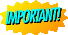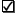Question #1: Css = ? Css = Q / ke * Vd where: Q = 150 mg/min ke = 0.046/hr and Vd = 40 L Css = 150 mg/min /((0.046/60min)*40,000ml)notice how the units are consistent: hr in ke changed to min to match Q (mg/min) and 40 L is represented as 40,000 mlAnswer: Css = 4.89 mg/ml   Question #2: Css = ? Css = Q / ke * Vd where: Q = 150 mg/min ke = 0.046/hr and Vd Css = 150 mg/min /((0.046/60min)*12,000ml)notice how the units are consistent: hr in ke changed to min to match Q (mg/min) and 12 L is represented as 12,000mlAnswer: Css = 16.23 ug/ml   Question #3: Css = ? Css = Q / ke * Vd where: Q = 300 mg/min ke = 0.046/hr and Vd 40 L so Css = 300 ug/min /((0.046/60min)*40,000ml)notice how the units are consistent: hr in ke changed to min to match Q (mg/min) and 40 L is represented as 40,000mlAnswer: Css = 9.74 mg/ml   Question #4: Css = ? Css = Q / ke * Vd where: Q = 450 m g/min ke = 0.046/hr and Vd 40 L> so Css = 450 mg/min /((0.046/60min)* 40,000ml)notice how the units are consistent: hr in ke changed to min to match Q (mg/min) and 40 Lis represented as 40,000mlAnswer: Css = 14.61 mg/ml   Question #5: f = 1 -e -ket this equation allows calculation of the time (t) need to reach a new steady-state concentration following a constant infusion of drug; f is the fraction of the shift from one steady state to the other. The question here asks for a 50% or 0.5 shift. so: f = 1 -e -ket or 0.5 = 1 - e-(0.046/hr)t or 0.5 = e(-0.046/hr)t now take antilogs: -0.693 = -0.046 t -0.693/-0.046 = tAnswer: t = 15 hours; note that a drug will always reach 50% of Css in one t1/2   Question #6: Q = ? Css = Q / ke * Vd where: Css = 25 ug/ml Q = ? m g/min ke = 0.046/hr and Vd = 40 L 25 ug/ml = Q / ((0.046/60 min) * 40,000 ml)Answer: Q = 760 ug/min   Question #7: f = 1 -e -ket this equation allows calculation of the time (t) need to reach a new steady-state concentration following a constant infusion of drug; f is the fraction of the shift from one steady state to the other. The question here asks for a 90% or 0.9 shift. so: f = 1 -e -ket or 0.9 = 1 - e-(0.046/hr)t or 0.1 = e(-0.046/hr)t now take antilogs: -2.3 = -0.046 t -2.3/-0.046 = tAnswer: t = 50 hours;